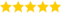# Init in Python

Last updated on 22nd Sep 2020, Artciles, Blog

Guna (Sr Technical Project Manager - Python )

He is a TOP-Rated Domain Expert with 11+ Years Of Experience, Also He is a Respective Technical Recruiter for Past 5 Years & Share's this Informative Articles For Freshers(5.0) | 14827 Ratings 597

### The __init__ method

There are many method names which have special significance in Python classes. We will see the significance of the __init__ method now.

The __init__ method is run as soon as an object of a class is instantiated. The method is useful to do any initialization you want to do with your object. Notice the double underscore both in the beginning and at the end in the name.

### Example

• #!/usr/bin/python
• # Filename: class_init.py
• class Person:
• def __init__(self, name):
• self.name = name
• def sayHi(self):
• print ‘Hello, my name is’, self.name
• p = Person(‘Swaroop’)
• p.sayHi()
• # This short example can also be written as Person(‘Swaroop’).sayHi()

### Output

\$ python class_init.py

Hello, my name is Swaroop

### How It Works

Here, we define the __init__ method as taking a parameter name (along with the usual self). Here, we just create a new field also called name. Notice these are two different variables even though they have the same name. The dotted notation allows us to differentiate between them.

Most importantly, notice that we do not explicitly call the __init__ method but pass the arguments in the parentheses following the class name when creating a new instance of the class. This is the special significance of this method.

Now, we are able to use the self.name field in our methods which is demonstrated in the sayHi method.

### Example 1:

• class A: def __init__(self): self.a=10

In the above example, we have created class A which consists of __init__(self) function. Here we are initializing

• self.a to 10 Object=A()

In the above code, we are calling an object for class A with name “Object” & we are using class reference A to access

__init__(self) which is defined in class A. This method is creating an object of class A named “Object” and calling

__init__(self) where ”self” in a reference to an object instance and it initializes self.a to 10

### __init__() with multiple arguments

__init__() function, 1 argument is always treated as a reference to an object and rest of arguments which are passed are consider as initialization parameters

### Syntax :

• __init__(<reference to an object >[,param1,param2…paramn]):
• <reference to an object>.<variable-name-param1>=param1
• <reference to an object>.<variable-name-param2>=param2 . . .
• <reference to an object>.<variable-name-paramn>=paramn

### Example 2:

• class A : def __init__(self, x, y): self.x=x self.y=y obj=A(10,20) obj.x obj.y

In the above example, we are passing x & y arguments along with first object reference ”self”. In __init__() function “x” and “y” are assigned to “self.x” and “self.y”

When we are creating an object of class A named “obj”, it is calling __init__() method via class constructor call “A(10,20)”.Here we are passing actual values “10” & “20” to __init__() methods arguments “x” & “y” respectively. We have defined __init__() method definition as below

• def __init__(self, x, y): self.x=x self.y=y

where , self .x = x =10  and self.y=y=20 , here initialization is achieved with multiple parameters. Let’s see more examples to understand in depth.

# Create class Human which will initialize values like “Height” and “Weight”

• class Human: def __init__(self): self.height=5.6 self.weight=65 obj = Human() print(obj.height) print(obj.weight)

### Output :

c:/users/421209/Documents/!Python/programs>A.py 5.6 65

# Create a class Rectangle which will take length and breadth values in the class constructor call